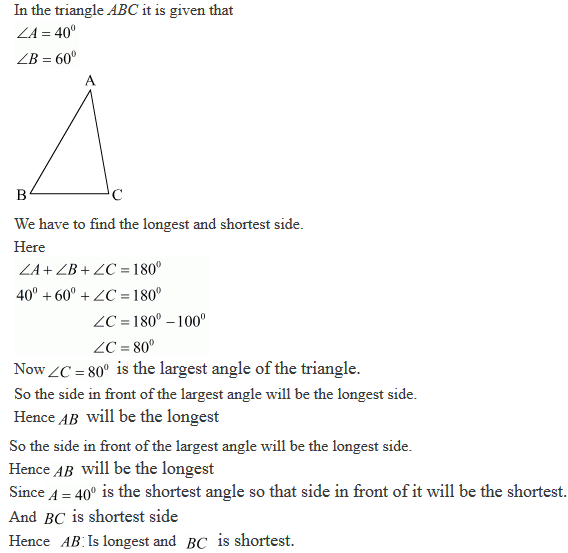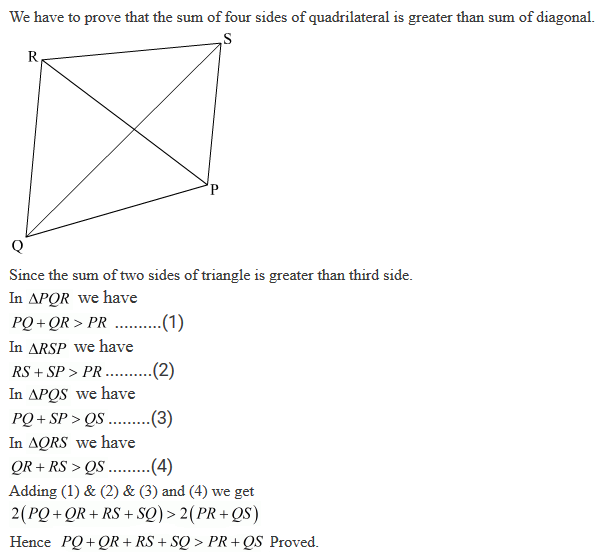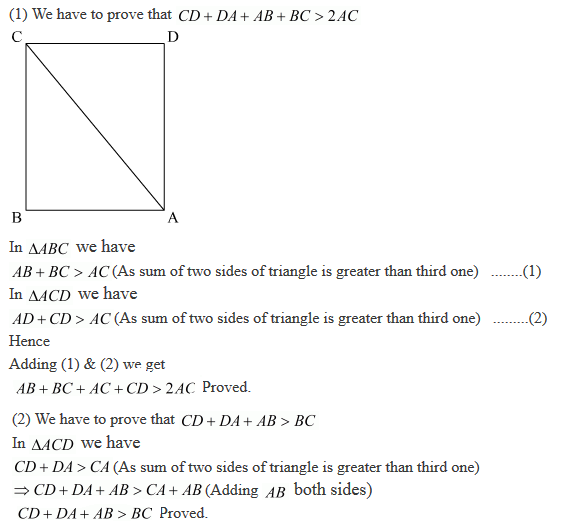#### Chapter 10 Congruent Triangles R.D. Sharma Solutions for Class 9th Exercise 10.6

Exercise 10.6

1.
1. In ΔABC, if ∠A = 40° and ∠B = 60°. Determine the longest and shortest sides of the triangle.

Solution2. In a ΔABC, if ∠B = ∠C = 45°, which is the longest side?

Solution3. In ΔABC, side AB is produced to D so that BD = BC. If ∠B = 60° and ∠A = 70°. Prove that:

Solution4. Is a possible to draw a triangle with sides of length 2 cm, 3 cm and 7 cm?

Solution

As we know that a triangle can only be formed if
The sum of two sides is greater than the third side.
Here we have 2 cm, 3 cm and 7 cm as sides.
If we add 2+3 = 5
5<7 (Since 5 is less than 7)
Hence, the sum of two sides is less than the third sides
So, the triangle will not exist.

5. In Δ ABC, ∠B = 35°, ∠C = 65° and the bisector of ∠BAC meets BC in P. Arrange AP, BP and CP in descending order.

Solution6. O is any point in the interior of △ABC. Prove that:
(i) AB+AC > OB+OC
(ii) AB + BC + CA > OA + OB + OC
(iii) OA + OB + OC > 1/2 (AB+BC+CA)

SolutionWe know that in a triangle the sum of any two sides is greater than the third side.
So, we have
In ΔABC
AB + BC > AC
BC + AC > AB
AC + AB > BC

In ΔOBC
OB + OC > BC …….(i)

In ΔOAC
OA + OC > AC ……(ii)

In ΔOAB
OA + OB > AB ……(iii)

Now, extend (or) produce BO to meet AC in D.

Now, in ΔABD, we have
AB + AD > BO + OD …….(iv) [BD = BO + OD]

Similarly in ΔODC, we have
OD + DC > OC ……(v)

(i) Adding (iv) and (v), we get

AB + AD + OD + DC > BO + OD + OC
AB + (AD + DC) > OB + OC
AB + AC > OB + OC ……(vi)

Similarly, we have
BC + BA > OA + OC ……(vii)
and CA+ CB > OA + OB ……(viii)

(ii) Adding equation (vi), (vii) and (viii), we get

AB + AC + BC + BA + CA + CB > OB + OC + OA + OC + OA + OB
⇒ 2AB + 2BC + 2CA > 2OA + 2OB + 2OC
⇒ 2(AB + BC + CA) > 2(OA + OB + OC)
⇒AB + BC + CA > OA + OB + OC

(iii) Adding equations (i), (ii) and (iii)

OB + OC + OA + OC + OA + OB > BC + AC + AB
2OA + 2OB + 2OC > AB + BC + CA
We get,
2(OA+OB+OC) > AB + BC +CA
(OA+OB + OC) > (1/2)(AB + BC +CA)

7. Prove that the perimeter of a triangle is greater than the sum of its altitudes.

Solution8. Prove that in a quadrilateral the sum of all the sides is greater than the sum of its diagonals.

Solution9. In Fig. 10.131, prove that:
(i) CD + DA + AB + BC > 2AC
(ii) CD + DA + AB > BCSolution10. Which of the following statements are true (T) and which are false (F)?

(i) Sum of the three sides of a triangle is less than the sum of its three altitudes.
(ii) Sum of any two sides of a triangle is greater than twice the median drawn to the third side.
(iii) Sum of any two sides of a triangle is greater than the third side.
(iv) Difference of any two sides of a triangle is equal to the third side.
(v) If two angles of a triangle are unequal, then the greater angle has the larger side opposite to it.
(vi) Of all the line segments that can be drawn from a point to a line not containing it, the perpendicular line segment is the shortest one.

Solution

(i) False
(ii) True
(iii) True
(iv) False
(v) True
(vi) True

11. Fill in the blanks to make the following statements true.
(i) In a right triangle the hypotenuse is the ...... side.
(ii) The sum of three altitudes of a triangle is ....... than its perimeter.
(iii) The sum of any two sides of a triangle is ..... than the third side.
(iv) If two angles of a triangle are unequal, then the smaller angle has the ..... side opposite to it.
(v) Difference of any two sides of a triangle is ...... than the third side.
(vi) If two sides of a triangle are unequal, then the larger side has ...... angle opposite to it.

Solution

(i) Largest
(ii) Less
(iii) Greater
(iv) Smaller
(v) Less
(vi) Greater